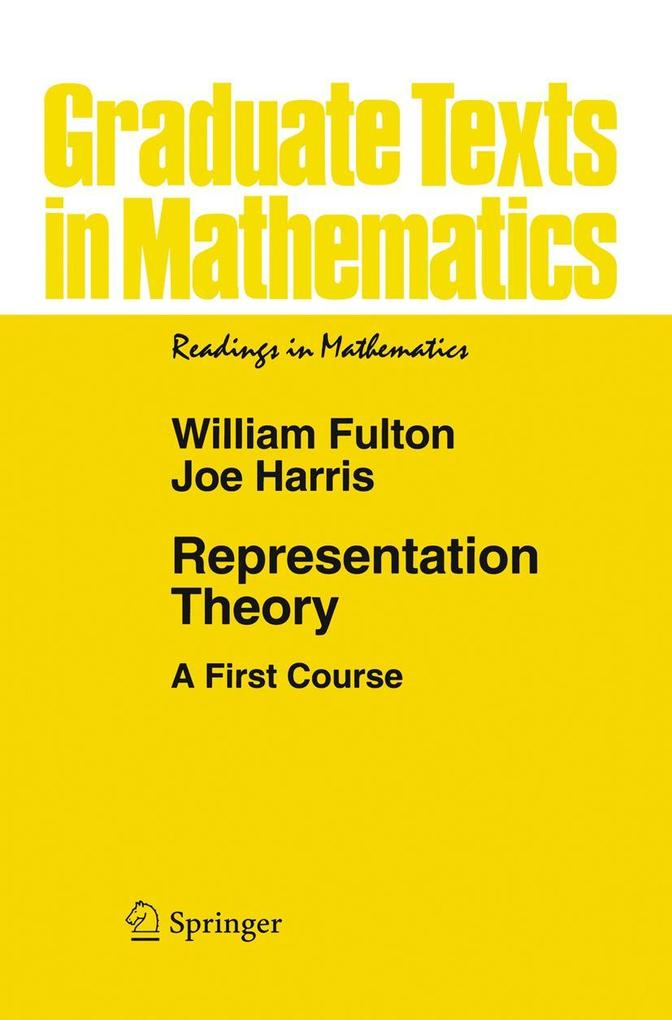Klick ins BuchPORTO-
FREI

# Representation Theory

A First Course.Lieferbar innerhalb von 3 bis 5 Werktagen
Buch (kartoniert)
Buch (kartoniert) € 63,99* inkl. MwSt.
Portofrei*
Dieser Artikel ist auch verfügbar als:

## Produktdetails

Titel: Representation Theory
Autor/en: William Fulton, Joe Harris

ISBN: 0387974954
EAN: 9780387974958
A First Course.
1st Corrected ed. 2004. Corr. 3rd printing 1999.
Paperback.
Sprache: Englisch.
Springer New York

30. Juli 1999 - kartoniert - 568 Seiten

The primary goal of these lectures is to introduce a beginner to the finite dimensional representations of Lie groups and Lie algebras. Since this goal is shared by quite a few other books, we should explain in this Preface how our approach differs, although the potential reader can probably see this better by a quick browse through the book. Representation theory is simple to define: it is the study of the ways in which a given group may act on vector spaces. It is almost certainly unique, however, among such clearly delineated subjects, in the breadth of its interest to mathematicians. This is not surprising: group actions are ubiquitous in 20th century mathematics, and where the object on which a group acts is not a vector space, we have learned to replace it by one that is {e. g. , a cohomology group, tangent space, etc. }. As a consequence, many mathematicians other than specialists in the field {or even those who think they might want to be} come in contact with the subject in various ways. It is for such people that this text is designed. To put it another way, we intend this as a book for beginners to learn from and not as a reference. This idea essentially determines the choice of material covered here. As simple as is the definition of representation theory given above, it fragments considerably when we try to get more specific.
I: Finite Groups.- 1. Representations of Finite Groups.-
1.1: Definitions.-
1.2: Complete Reducibility; Schur's Lemma.-
1.3: Examples: Abelian Groups;
$${\mathfrak{S}_3}$$.- 2. Characters.-
2.1: Characters.-
2.2: The First Projection Formula and Its Consequences.-
2.3: Examples:
$${\mathfrak{S}_4}$$and
$${\mathfrak{A}_4}$$.-
2.4: More Projection Formulas; More Consequences.- 3. Examples; Induced Representations; Group Algebras; Real Representations.-
3.1: Examples:
$${\mathfrak{S}_5}$$
and
$${\mathfrak{A}_5}$$.-
3.2: Exterior Powers of the Standard Representation of
$${\mathfrak{S}_d}$$.-
3.3: Induced Representations.-
3.4: The Group Algebra.-
3.5: Real Representations and Representations over Subfields of
$$\mathbb{C}$$.- 4. Representations of:
$${\mathfrak{S}_d}$$
Young Diagrams and Frobenius's Character Formula.-
4.1: Statements of the Results.-
4.2: Irreducible Representations of
$${\mathfrak{S}_d}$$.-
4.3: Proof of Frobenius's Formula.- 5. Representations of
$${\mathfrak{A}_d}$$
and
$$G{L_2}\left( {{\mathbb{F}_q}} \right)$$.-
5.1: Representations of
$${\mathfrak{A}_d}$$.-
5.2: Representations of
$$G{L_2}\left( {{\mathbb{F}_q}} \right)$$
and
$$S{L_2}\left( {{\mathbb{F}_q}} \right)$$.- 6. Weyl's Construction.-
6.1: Schur Functors and Their Characters.-
6.2: The Proofs.- II: Lie Groups and Lie Algebras.- 7. Lie Groups.-
7.1: Lie Groups: Definitions.-
7.2: Examples of Lie Groups.-
7.3: Two Constructions.- 8. Lie Algebras and Lie Groups.-
8.1: Lie Algebras: Motivation and Definition.-
8.2: Examples of Lie Algebras.-
8.3: The Exponential Map.- 9. Initial Classification of Lie Algebras.-
9.1: Rough Classification of Lie Algebras.-
9.2: Engel's Theorem and Lie's Theorem.-
9.3: Semisimple Lie Algebras.-
9.4: Simple Lie Algebras.- 10. Lie Algebras in Dimensions One, Two, and Three.-
10.1: Dimensions One and Two.-
10.2: Dimension Three, Rank 1.-
10.3: Dimension Three, Rank 2.-
10.4: Dimension Three, Rank 3.- 11. Representations of
$$\mathfrak{s}{\mathfrak{l}_2}\mathbb{C}$$.-
11.1: The Irreducible Representations.-
11.2: A Little Plethysm.-
11.3: A Little Geometric Plethysm.- 12. Representations of
$$\mathfrak{s}{\mathfrak{l}_3}\mathbb{C},$$
Part I.- 13. Representations of
$$\mathfrak{s}{\mathfrak{l}_3}\mathbb{C},$$
Part II: Mainly Lots of Examples.-
13.1: Examples.-
13.2: Description of the Irreducible Representations.-
13.3: A Little More Plethysm.-
13.4: A Little More Geometric Plethysm.- III: The Classical Lie Algebras and Their Representations.- 14. The General Set-up: Analyzing the Structure and Representations of an Arbitrary Semisimple Lie Algebra.-
14.1: Analyzing Simple Lie Algebras in General.-
14.2: About the Killing Form.- 15.
$$\mathfrak{s}{\mathfrak{l}_4}\mathbb{C}$$
and
$$\mathfrak{s}{\mathfrak{l}_n}\mathbb{C}$$.-
15.1: Analyzing
$$\mathfrak{s}{\mathfrak{l}_n}\mathbb{C}$$.-
15.2: Representations of
$$\mathfrak{s}{\mathfrak{l}_4}\mathbb{C}$$
and
$$\mathfrak{s}{\mathfrak{l}_n}\mathbb{C}$$.-
15.3: Weyl's Construction and Tensor Products.-
15.4: Some More Geometry.-
15.5: Representations of
$$G{L_n}\mathbb{C}$$.- 16. Symplectic Lie Algebras.-
16.1: The Structure of
$$S{p_{2n}}\mathbb{C}$$
and
$$\mathfrak{s}{\mathfrak{p}_2n}\mathbb{C}$$.-
16.2: Representations of
$$\mathfrak{s}{\mathfrak{p}_4}\mathbb{C}$$.- 17.
$$\mathfrak{s}{\mathfrak{p}_6}\mathbb{C}$$
and
$$\mathfrak{s}{\mathfrak{p}_2n}\mathbb{C}$$.-
17.1: Representations of
$$\mathfrak{s}{\mathfrak{p}_6}\mathbb{C}$$.-
17.2: Representations of
$$\mathfrak{s}{\mathfrak{p}_2n}\mathbb{C}$$
in General.-
17.3: Weyl's Construction for Symplectic Groups.- 18. Orthogonal Lie Algebras.-
18.1:
$$S{O_m}\mathbb{C}$$
and
$$\mathfrak{s}{\mathfrak{o}_m}\mathbb{C}$$.-
18.2: Representations of
$$\mathfrak{s}{\mathfrak{o}_3}\mathbb{C},$$$$\mathfrak{s}{\mathfrak{o}_4}\mathbb{C},$$
and

\mathfrak{s}{\mathfrak{o}_5}\mathbb
Kundenbewertungen zu William Fulton, Joe… „Representation Theory“
Noch keine Bewertungen vorhanden
Zur Rangliste der Rezensenten
Veröffentlichen Sie Ihre Kundenbewertung:
Entdecken Sie mehr
Unsere Leistungen auf einen Klick
Unser Service für Sie
Zahlungsmethoden
Bequem, einfach und sicher mit eBook.de. mehr Infos akzeptierte Zahlungsarten: Überweisung, offene Rechnung,
Visa, Master Card, American Express, Paypal mehr Infos
Geprüfte Qualität
• Datenschutz
• Sichere Zahlung
• SSL-Verschlüsselung
Servicehotline
+49 (0)40 4223 6096
Mo. - Fr. 8.00 - 20.00 Uhr
Sa. 10.00 - 18.00 Uhr
Chat
Ihre E-Mail-Adresse eintragen und kostenlos informiert werden:
2 Diese Artikel unterliegen nicht der Preisbindung, die Preisbindung dieser Artikel wurde aufgehoben oder der Preis wurde vom Verlag gesenkt. Die jeweils zutreffende Alternative wird Ihnen auf der Artikelseite dargestellt. Angaben zu Preissenkungen beziehen sich auf den vorherigen Preis.

3 Ihr Gutschein AUDIO20 gilt bis einschließlich 30.04.2020 und nur für die Kategorie Hörbuch Downloads und Hörbuch CDs. Der Gutschein kann mehrmals eingelöst werden. Sie können den Gutschein ausschließlich online einlösen unter www.ebook.de. Der Gutschein ist nicht mit anderen Gutscheinen und Geschenkkarten kombinierbar. Eine Barauszahlung ist nicht möglich. Ein Weiterverkauf und der Handel des Gutscheincodes sind nicht gestattet.

4 Der gebundene Preis dieses Artikels wird nach Ablauf des auf der Artikelseite dargestellten Datums vom Verlag angehoben.

5 Der Preisvergleich bezieht sich auf die unverbindliche Preisempfehlung (UVP) des Herstellers.

6 Der gebundene Preis dieses Artikels wurde vom Verlag gesenkt. Angaben zu Preissenkungen beziehen sich auf den vorherigen Preis.

7 Die Preisbindung dieses Artikels wurde aufgehoben. Angaben zu Preissenkungen beziehen sich auf den vorherigen Preis.

* Alle Preise verstehen sich inkl. der gesetzlichen MwSt. Informationen über den Versand und anfallende Versandkosten finden Sie hier.

eBook.de - Meine Bücher immer dabei
eBook.de ist eine Marke der Hugendubel Digital GmbH & Co. KG
Folgen Sie uns unter: